# OliNo

Renewable Energy

## iON LED – ION-TUEXAB15025-F4-0515iON LED presents an LED tube. The measurements from OliNo show that the lamp emits a warm/neutral white light with a color temperature of 3989 K. The lamp consumes 23.7 W and has a luminous flux of 2684 lm. This results in an efficacy of 113 lm/W. The lamps energy category label is A+.

This article shows the measurement results. Many parameters are also found in the Eulumdat file.

See this overview for a comparison with other light bulbs.

### Summary measurement data

parameter meas. result remark
Color temperature 3989 K warm/neutral white
Luminous intensity Iv 690.2 Cd Measured straight underneath the lamp.
Illuminance modulation index 34 % Measured with a light sensor looking at the lamp (angle not defined). Is a measure for the amount of flickering.
Beam angle 142 deg 142 deg is the beam angle for the C0-C180-plane (perpendicular to the length direction of the lamp) and 109 deg is the beam angle for the C90-C270 plane, which is along the length direction of the lamp.
Power P 23.7 W The net power consumed.
Power Factor 0.94 An electrical load with this power factor means that for every 1 kWh net energy consumed, there has been 0.35 kVAhr for reactive energy.
THD 12 % Total Harmonic Distortion.
Max inrush current 0.825 A This current has been found at a voltage start angle of 80 degrees.
Luminous flux 2684 lm Measured with photogoniometer, calculation done as described in LM79-08.
Luminous efficacy 113 lm/W
EU2013-label classification A+ The energy class, from A++ (more efficient) to E (least efficient). This label is an update of the previous version, and compulsory from Sept 2013.
CRI_Ra 83 Color Rendering Index.
Qa_CQS 83.5 QCS (v9.0.3) is an improved indicator (over CRI) of how well colors are rendered.
Qg_CQS 0.95 Gamut Area Ratio.
Coordinates chromaticity diagram x=0.3794 en y=0.3816
Fitting FL-tube This tube is connected from one side to the grid voltage.
PAR-value 6.3 uMol/s/m2 The number of photons seen by an average plant when it is lit by the light of this light bulb. Value valid at 1 m distance from light bulb.
PAR-photon efficacy 1.0 uMol/s/We The total emitted number of photons by this light, divided by its consumption in W. It indicates a kind of efficacy in generating photons.
Photon current 39.9 uMol/s The total number of photons in the light of this lamp.
S/P ratio 1.7 This factor indicates the amount of times more efficient the light of this light bulb is perceived under scotopic circumstances (low environmental light level).
L x W x H external dimensions 1500 mm x 28 mm x 32 mm External dimensions of the lamp.
L x W x H luminous area 1460 mm x 26 mm x 13 mm Dimensions of the luminous area (used in Eulumdat file). It is the surface of the matte white cover.
General remarks The ambient temperature during the whole set of illuminance measurements was 25.5 – 26.2 deg C.

The temperature of the lamp gets maximally about 9.5 degrees hotter than ambient temperature.

Warm up effect: During the warmup time the illuminance varies during 11 minutes and decreases with 5 %.
During the warmup time the power doesn’t vary significantly (< 5 %).
The variation in efficacy (calculated as indication by simplydividing the illuminance by the power) during the warming up is -3 %. A very high negative value indicates a significant decrease for instance due to heating up of the lamp (decrease of lifetime).

Voltage dependency: There is no (significant) dependency of the illuminance when the power voltage varies between 200 – 250 V AC.
There is no (significant) dependency of the consumed power when the power voltage varies between 200 – 250 V AC.

At the end of the article an additional photo.

Eff-variation -3 % This is the variation in efficacy (calculated as indication by simply dividing the illuminance by the power) during the warming up. A very high negative value indicates a significant decrease for instance due to heating up of the lamp (decrease of lifetime).
Dimmable no Info from manufacturer.

Biologic effect factor
0.533 According to pre-norm DIN V 5031-100:2009-06.
Blue Light Hazard risk group 0 0=exempt, 1=low, 2 = moderate, 3=high risk.
form factor tube
Eulumdat fileRight click on icon and save the file.
IES fileRight click on icon and save the file.

### Overview table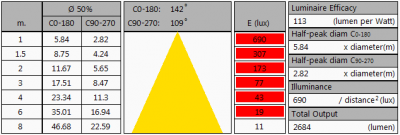Please note that this overview table makes use of calculations, use this data with care as explained on the OliNo site. E (lux) values are not accurate, when within 5 x 1460 mm (maximal luminous size, eventually diagonally measured)= 7300 mm. Within this distance from the lamp (data given in red), the measured lux values will be less than the computed values in this overview as the measurements are then within the near field of the lamp.

### EU 2013 Energy label classification

Since Sept 2013 these labels will be needed.

Important for the energy classification are the corrected rated power and the useful luminous flux.
The measured rated power is 23.7 W and might need to be corrected. The correction is dependent from the lamp type and whether or not the lamp control gear is included or not. The choice for this lamp is the following classification: Lamps with own control gear (external or internal). As a result the corrected rated power becomes: 23.7 W.
The luminous flux measured is 2684 lm. The classification of this lamp needed to determine the useful flux is: Non-directional lamps. Then the useful flux becomes 2684 lm. Now a reference power can be calculated.

The energy efficiency coefficient is P_corr / P_ref = 0.12.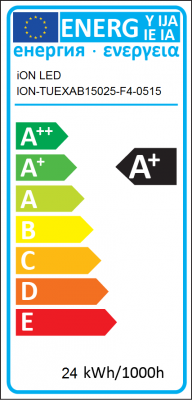EU energy label for this lampZip file with 6 EU energy labels of this lampThe lamp’s performance in the lumen-Watt field, with the energy efficacy fields indicated.

### Eulumdat light diagram

This light diagram below comes from the program Qlumedit, that extracts these diagrams from an Eulumdat file.

VOEG LICHTDIAGRAMPLAATJE IN

The light diagram giving the radiation pattern.

The light diagram indicates the beam in the C0-C180 plane (perpendicular to the length direction of the lamp) and in the plane perpendicular to that, the C90-C270 plane (along the length direction of the lamp).

### Illuminance Ev at 1 m distance, or luminous intensity Iv

Herewith the plot of the averaged luminous intensity Iv as a function of the inclination angle with the light bulb.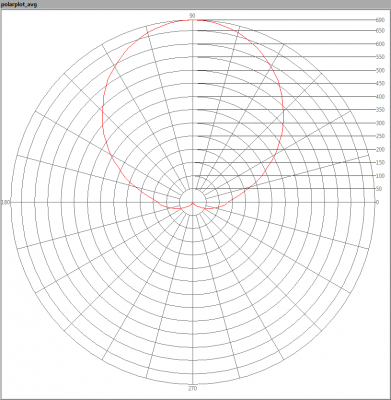The radiation pattern of the light bulb.

This radiation pattern is the average of the light output of the light diagram given earlier. Also, in this graph the luminous intensity is given in Cd.

These averaged values are used (later) to compute the lumen output.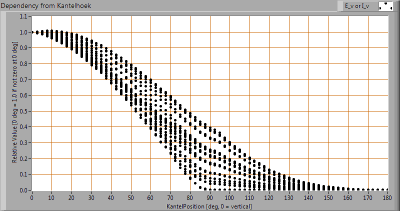Intensity data of every measured turn angle at each inclination angle.

This plot shows per inclination angle the intensity measurement results for each turn angle at that inclination angle. There normally are differences in illuminance values for different turn angles. However for further calculations the averaged values will be used.

When using the average values per inclination angle, the beam angle can be computed, being 142 deg for the C0-C180 plane and 109 deg for the C90-C270 plane.

### Luminous flux

With the averaged illuminance data at 1 m distance, taken from the graph showing the averaged radiation pattern, it is possible to compute the luminous flux.

The result of this computation for this light spot is a luminous flux of 2684 lm.

### Luminous efficacy

The luminous flux being 2684 lm, and the consumed power of the lamp being 23.7 Watt, results in a luminous efficacy of 113 lm/Watt.

### Electrical properties

The power factor is 0.94. An electrical load with this power factor means that for every 1 kWh net energy consumed, there has been 0.35 kVAhr for reactive energy.

 Lamp voltage 230.15 V Lamp current 0.109 A Power P 23.7 W Apparent power S 25.1 VA Power factor 0.94

Of this lamp the voltage across and the resulting current through it are measured and graphed.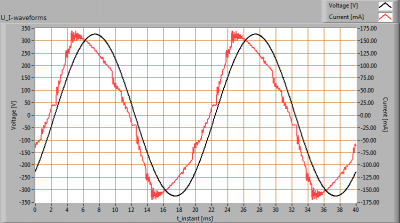Voltage across and current through the lightbulb

This current waveform has been checked on requirements posed by the norm IEC 61000-3-2:2006 (including up to A2:2009).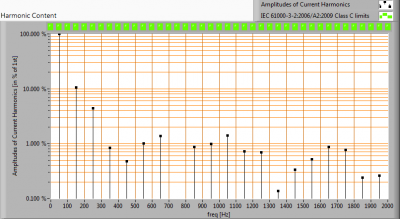Harmonics in the current waveform and checked against IEC61000-3-2:2006 and A2:2009

When the consumed power is <= 25 W there are no limits for the harmonics.

The Total Harmonic Distortion of the current is computed and its value is 12 %.

### Inrush current

The inrush current has been measured for different voltage start angles; from 0 – 170 degrees with a 10 degrees step. The current- and voltage values have been acquires at a sample speed of 39.9 kS/s. Then this data has been fed into a second order 2kHz low pass Butterworth filter. This removes the current spikes that do not represent relevant values.
The lamp was two minutes off before every inrush current measurement was made.

 Test voltage 230.0 V Frequency of the voltage 50.0 Hz Maximum inrush current 0.825 A This current has been found at a voltage start angle of 80 degrees. Pulse width of max inrush current 3.3E-4 s This is the time that the pulse is higher than 10 % of the max inrush current. Minimal inrush current 0.045 A This current has been found at a voltage start angle of 0 degrees. I^2 x t after 10 ms at 0 deg voltage start angle 6.000E-6 A This is the I^2 t value when a zero crossing detector is used to start the voltage from 0 degrees.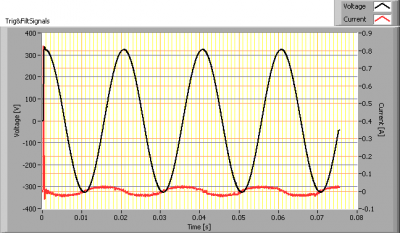Inrush current found at worst-case voltage start angle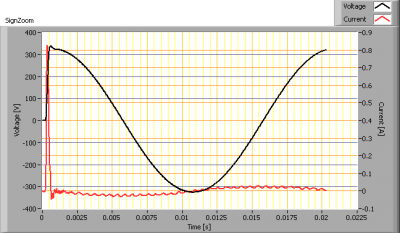First cycle of the maximum inrush current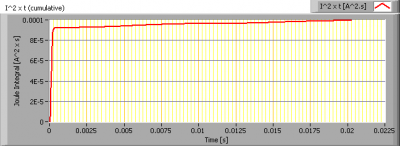The energy I2t during the first 10 ms of the first current cycle

### Temperature measurements lamp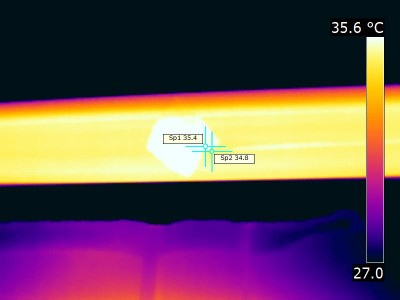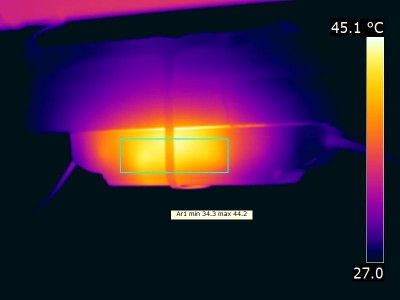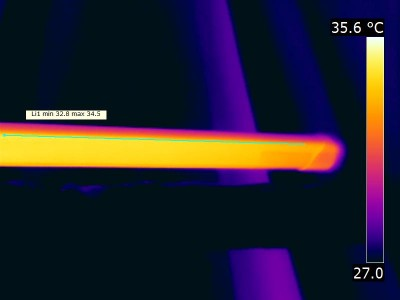Temperature image(s).

 status lamp > 2 hours on ambient temperature 26.3 deg C reflected background temperature 26.3 deg C camera Flir T335 emissivity 0.90 (metal), 0.95 measurement distance 0.5, 1 m IFOVgeometric 0.136 mm per 0.1 m distance NETD (thermal sensitivity) 50 mK

### Color temperature and Spectral power distribution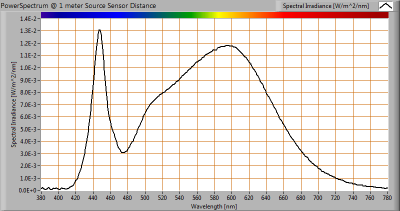The spectral power distribution of this light bulb, energies on y-axis valid at 1 m distance.

The measured color temperature is 3989 K which is warm/neutral white.

This color temperature is measured straight underneath the light bulb. Below a graph showing the color temperature for different inclination angles.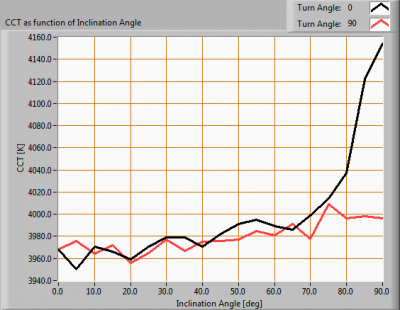Color temperature as a function of inclination angle.<%2